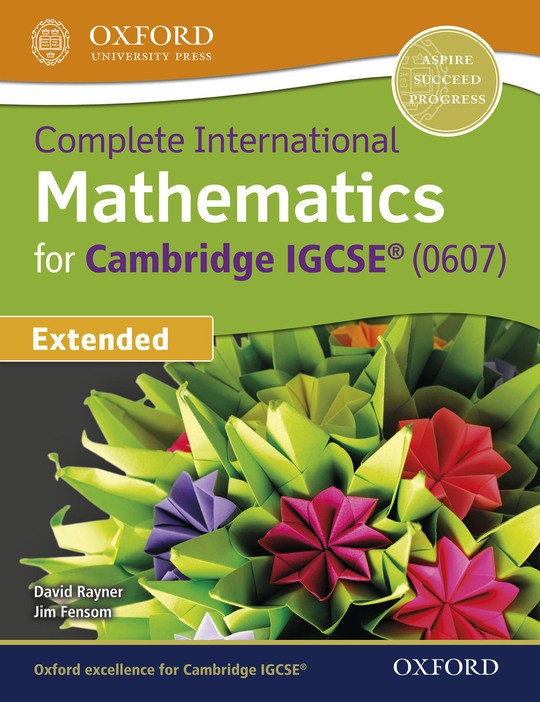\$42.50

# Complete International Mathematics for Cambridge IGCSE® Extended

By David Rayner, Jim Fensom
US\$ 42.50
The publisher has enabled DRM protection, which means that you need to use the BookFusion iOS, Android or Web app to read this eBook. This eBook cannot be used outside of the BookFusion platform.
Book Description

An expert dual approach - with David Rayner injecting his well-known rigour and exact match to the Cambridge IGCSE® (0607) specification, plus IB Maths specialist Jim Fensom incorporating the enrichment and IB-focus needed for success in future IB study. The resource is fully up-to-date and matched to the latest Cambridge syllabus. It provides thorough exam support, with a practice-based approach and plenty of worked examples to reinforce understanding. it
includes free access to online resources focused on building assessment confidence, with revision help and a whole suite of past exam papers. This resource offers just the right amount of rigour for Cambridge IGCSE International Mathematics and is specially tailored for IB preparation, with integrated graphics
calculator guidance providing invaluable support. A solid foundation which will sufficiently challenge students to hit the ground running in IB Maths.

• Front Cover
• Title Page
• Contents
• Introduction
• What’s on the website?
• 1 Using your graphic display calculator
• 1 Using templates in basic calculations
• 1.1 Fractions
• 1.2 Square roots
• 1.3 n th roots
• 1.4 Exponents
• 1.5 Absolute value
• 1.6 Logarithms
• 2 Working with graphs
• 2.1 Entering a function and choosing a window
• 2.2 Producing a table of values for a function
• 2.3 Finding zeros (roots)
• 2.4 Finding a local minimum
• 2.5 Finding a local maximum
• 2.6 Finding the intersection point of two graphs
• 3 Working with data
• 3.1 Calculation of basic statistics from a list
• 3.2 Calculation of basic statistics from a frequency table
• 3.3 Finding a linear regression equation
• 3.4 Drawing a scatter graph and the graph of the equation of linear regression
• 2 Number
• 2.1 Vocabulary and notation for sets of numbers
• 2.2 Arithmetic
• 2.3 Number facts and sequences
• 2.4 Approximations and estimation
• 2.5 Standard form
• 2.6 Ratio and proportion
• 2.7 Percentages
• 2.8 Speed, distance and time
• 3 Algebra 1
• 3.1 Negative numbers
• 3.2 Directed numbers
• 3.3 Formulae and expressions
• 3.4 Brackets and simplifying
• 3.5 Linear equations
• 3.6 Problems solved by linear equations
• 3.7 Simultaneous equations
• 3.8 Problems solved by simultaneous equations
• 3.9 Factorising
• 3.11 Problems solved by quadratic equations
• 4 Mensuration
• 4.1 Area
• 4.2 The circle
• 4.3 Arc length and sector area
• 4.4 Chord of a circle
• 4.5 Volume
• 4.6 Surface area
• 5 Functions 1
• 5.1 Function notation
• 5.2 Inverse function
• 5.4 Sketch graphs
• 5.5 Interpreting graphs
• 6 Investigations and mathematical modelling
• 6.1 Investigations
• 6.2 Mathematical modelling
• 7 Geometry
• 7.1 Fundamental results
• 7.2 Pythagoras’ theorem
• 7.3 Symmetry
• 7.4 Similarity
• 7.5 Circle properties
• 8 Algebra 2
• 8.1 Inequalities
• 8.2 Indices
• 8.3 Rearrangement and evaluation of formulae
• 8.4 Algebraic fractions
• 8.5 Difference method, n th term of a sequence
• 8.6 Geometric series
• 8.7 Variation, direct and inverse
• 9 Functions 2
• 9.1 Drawing and using graphs
• 9.2 Gradient of a line
• 9.3 The forms y = mx + c and ax + by = d
• 9.4 Curved graphs
• 9.5 Graphical solution of equations
• 9.6 Transformations of the graph of y = f(x)
• 9.7 Logarithm function
• 10 Trigonometry
• 10.1 Right-angled triangles
• 10.2 Sine, cosine and tangent for 0°, 30°, 45°, 60°, 90°
• 10.3 Three-dimensional problems
• 10.4 Sine, cosine, tangent for any angle
• 10.5 The sine rule
• 10.6 The cosine rule
• 11 Vectors and transformations
• 11.1 Vectors
• 11.2 Column vectors
• 11.3 Simple transformations
• 11.4 Combined transformations
• 12 Sets
• 12.1 Set notation
• 12.2 Logical problems
• 13 Probability
• 13.1 Probability
• 13.2 Mutually exclusive and independent events
• 13.3 Tree diagrams
• 14 Statistics
• 14.1 Reading and interpretation of data or graphs
• 14.2 Averages, range and quartiles
• 14.3 Histograms
• 14.4 Cumulative frequency
• 14.5 Scatter diagrams
• 15 Investigations and mathematical modelling 2
• 15.1 Investigations
• 15.2 Mathematical modelling
• 2 Number
• 3 Algebra 1
• 4 Mensuration
• 5 Functions 1
• 6 Investigations and mathematical modelling
• 7 Geometry
• 8 Algebra 2
• 9 Functions 2
• 10 Trigonometry
• 11 Vectors and transformations
• 12 Sets
• 13 Probability
• 14. Statistics
• 15 Investigations and mathematical modelling 2
• Index
• Back Cover
You May Also Like
Complete Mathematics for Cambridge IGCSE® Core
By David Rayner, Ian Bettison, Mathew Taylor
Complete Mechanics for Cambridge International AS & A Level
By Phillip Crossley, Martin Burgess, Jim Fensom
Complete Mathematics for Cambridge IGCSE® Extended
By David Rayner, Ian Bettison, Mathew Taylor
MYP Mathematics 4&5 Extended
By Rose Harrison, Clara Huizink, Aidan Sproat-Clements, Marlene Torres-Skoumal
Oxford International AQA Examinations: International GCSE Mathematics Core
By June Haighton, Steve Lomax, Steve Fearnley, Peter Mullarkey, Matt Nixon, James Nicholson